1. 解析输入函数f之后，分别生成∂f/∂x和∂f/∂y两个偏导数，然后将这三个二元函数转化为合法的OpenCL表达式。
2. 用OpenCL实现牛顿迭代法。
3. 将图像上的每一点分派到一个OpenCL线程，然后由无数并行的OpenCL线程计算自己的点。

 fp_t func(fp_t x, fp_t y) { return {动态生成}; } fp_t df_dx(fp_t x, fp_t y) { return {动态生成}; } fp_t df_dy(fp_t x, fp_t y) { return {动态生成}; } fp_t solvex(fp_t start, const fp_t consty) { for (int i = 0; i < MAX_ITER; ++i) { fp_t result = func(start, consty); if (result <= EPSILON && result >= -EPSILON) { return start; } fp_t d = df_dx(start, consty); if (d <= EPSILON && d >= -EPSILON) { return NAN; } else { start -= result / d; } } return NAN; } kernel void ComputeX( global write_only fp_t* points, int unit, int width, int cx, int cy, float origin_x, float origin_y) { int gx = get_global_id(0); int gy = get_global_id(1); uint write_loc = gx + gy * width; fp_t py = origin_y + (fp_t)(gy + 1 - cy) / unit; fp_t px = origin_x + (fp_t)(cx - gx - 1) / unit; points[write_loc] = solvex(px, py); }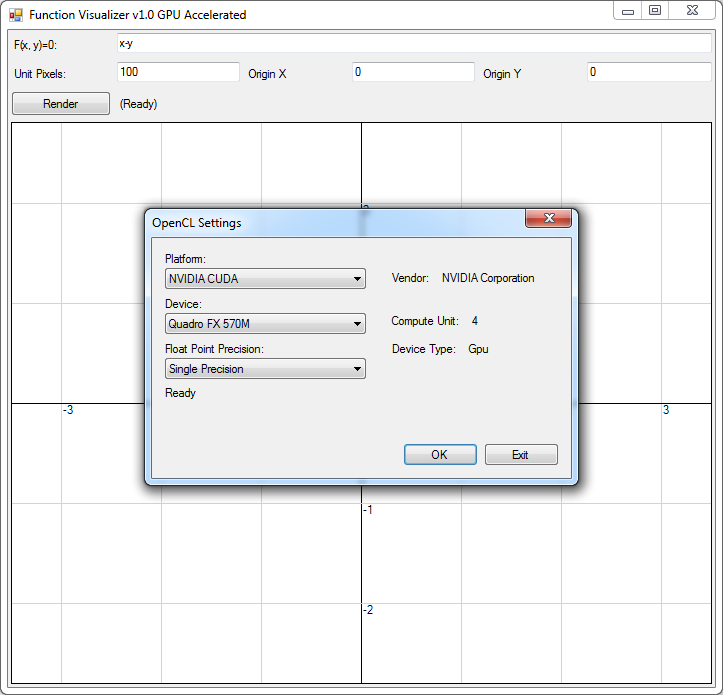AMD APP平台界面示例：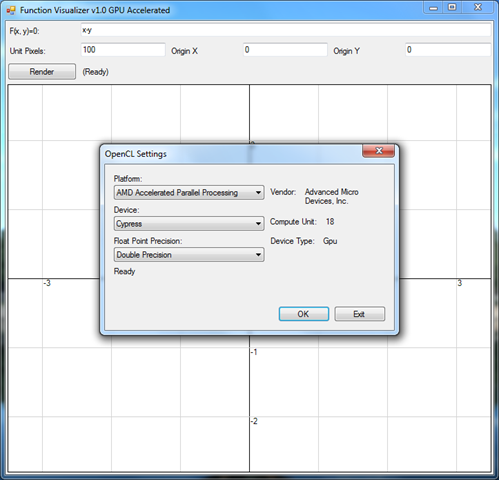Intel OpenCL平台界面示例：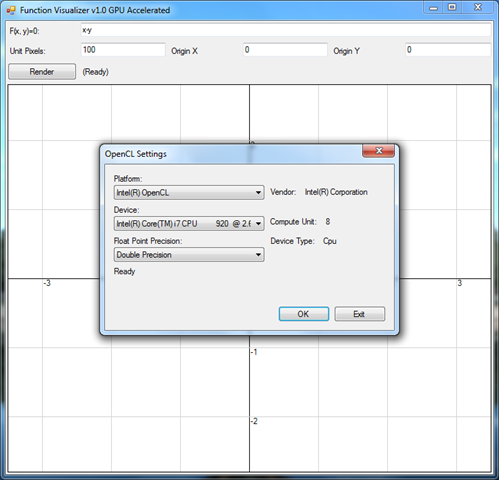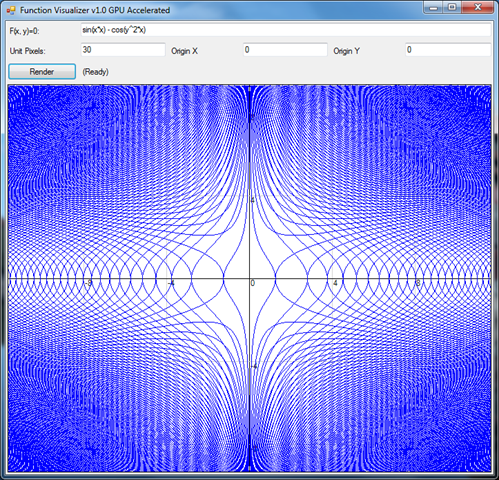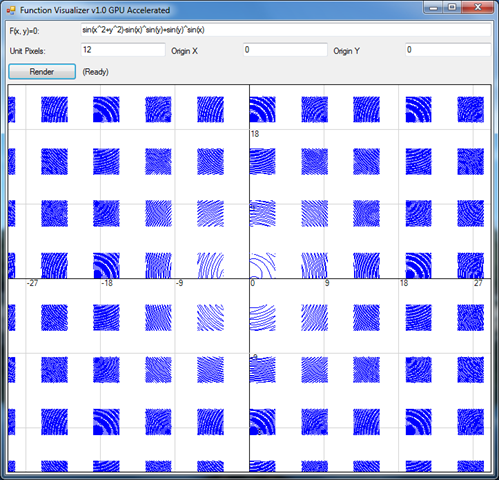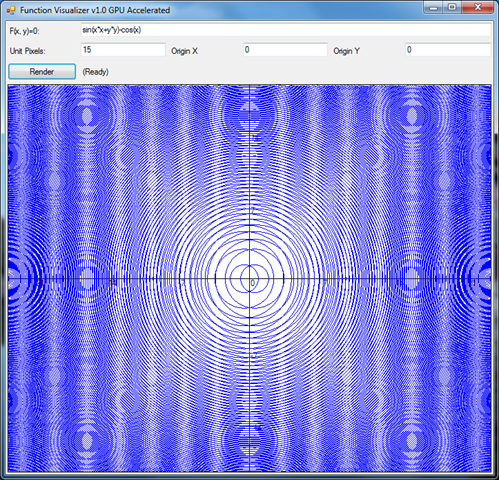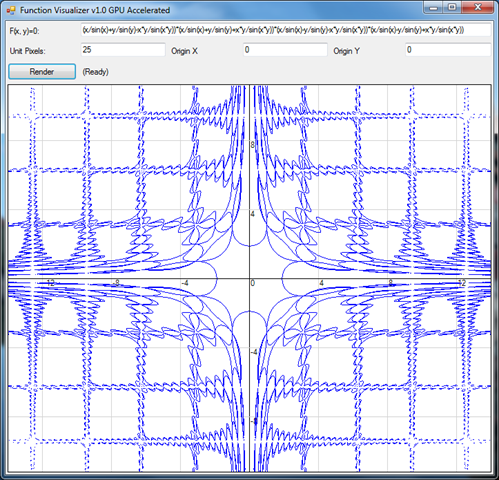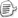posted on 2011-08-18 23:48 装配脑袋 阅读(...) 评论(...) 编辑 收藏